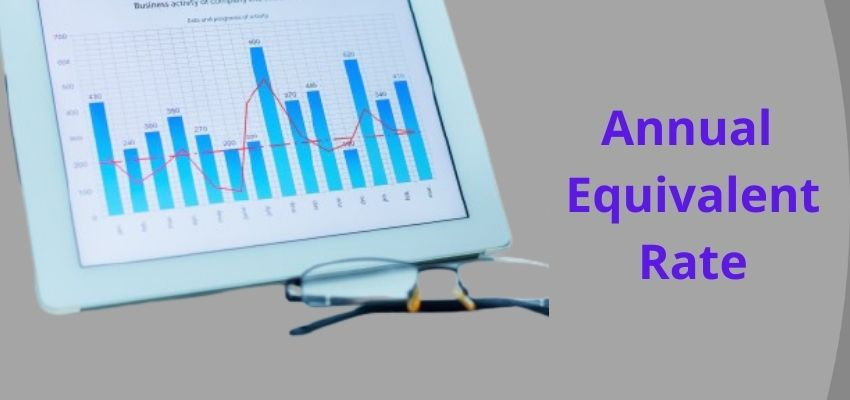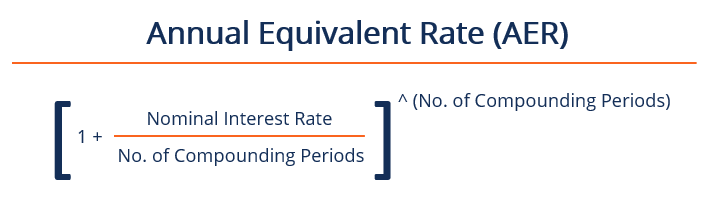# What is the Annual Equivalent Rate (AER)?

• AccountingAnnual Equivalent Rate or AER is the rate of interest an investor gets for a saving account for a year on a yearly basis. Annual Equivalent Rate or AER is a figure which shows what the interest rate on an account would be if interest was paid for a full year and compounded. The interest is calculated to determine the returns that a person can get by adding the interest payment to the amount originally deposited and the next interest payment will be established on the marginally higher account balance.

Some key factors of Annual Equivalent Rate:

• The AER is the rate an investor can expect to earn from an investment after taking compounding into account.
• AER is also known as the effective annual rate (EAR) or the annual percentage yield (APY).
• The AER will be higher than the stated or nominal rate if there is more than one compounding period a year. The spread between the two will grow greater with more compounding periods.

The formula for AER is:

(1 + i/n)n – 1

Where:

i = the stated annual interest rate

n = the number of compounding periods in one year## How to calculate Annual Equivalent Rate(AER)?

1. Divide the gross interest rate by the number of times a year that interest is paid and add one.
2. Raise the result to the number of times a year that interest is paid.
3. Subtract one from the subsequent result.

The AER is displayed as a percentage (%).

## Why we use Annual Equivalent Rate(AER)

The annual equivalent rate is used to compare the interest rates between loans or investments or saving deposits of different compounding periods, such as weekly, monthly, half-yearly, or yearly. Therefore, AER can be used by both individuals who are looking for the best savings account or an investor comparing bond yields.

The AER allows borrowers to see through the complications of loans and other products in which the compounding of the interest is worked out at intervals other than a year, such as weekly, monthly, or quarterly. This helps us to give borrowers greater clarity about the cost of the credit, known as the nominal interest rate. The AER recalculates the interest rate by figuring out what the total cost of the loan would be if it was structured in a conventional way, with interest compounded annually. It should not be confused with the Annual Percentage Rate (APR).

## 3 Limitations of Annual Equivalent Rate(AER)

1. Tax on interest : AER does not take account of any tax, you might have to pay the interest you earn. Most people won’t need to pay any tax on savings because of their tax-free Personal Savings Allowance, but high earners or big savers who exceed their allowance will need to take account of tax when comparing savings options.
2.  Don’t assume you will get the advertised AER : Banks and building societies often attract new savers with high-interest rates but may pay less to people who have had their savings account for a while.  Providers must communicate the actual rate they will receive.
3.  Long-term savings: Review the AER regularly, because it could become less competitive over time.  Switching accounts might give you a better return.